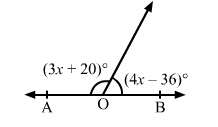# In the adjoining figure, what value of x will make AOB a straight line?

Question:

In the adjoining figure, what value of x will make AOB a straight line?Solution:

AOB will be a straight line if

$3 x+20+4 x-36=180^{\circ}$

$\Rightarrow 7 x=196^{\circ}$

$\Rightarrow x=28^{\circ}$

Hence, x = 28 will make AOB a straight line.